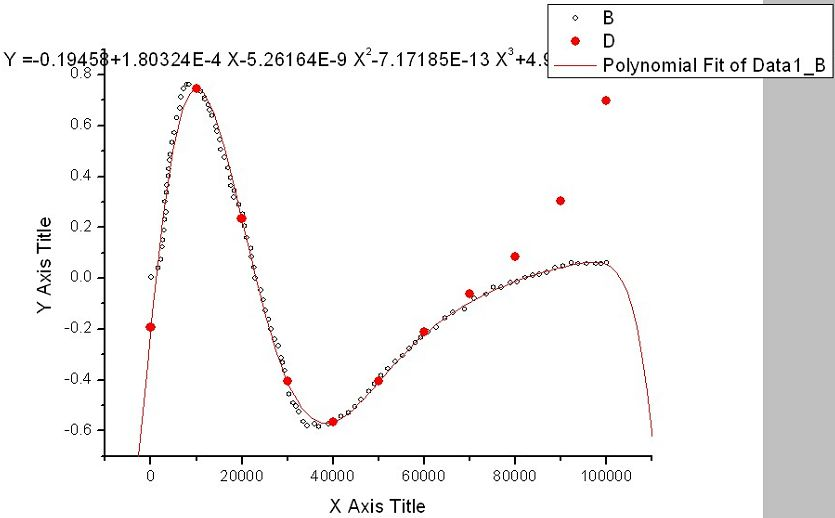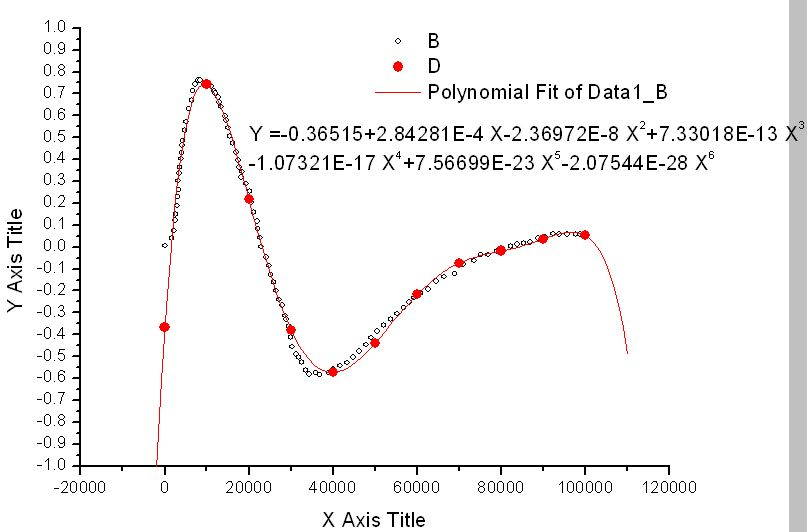# 关于Origin的多项式拟合三月 27, 201522:23:00 评论 1,506 views

Origin软件提供了多种非线性拟合工具，可以在“分析”菜单栏下找到。其中的多项式拟合对于许多不规则的函数曲线有比较好的拟合功能，一般需要选择合适的阶数才能达到比较好的拟合效果。下面针对一个实际例子，说明在应用多项式拟合功能时需要注意的问题。以某两列数据为例（好友梅子提供），其图形如下图：Y =-0.19458+1.80324E-4*X-5.26164E-9*X^2-7.17185E-13*X^3+4.99335E-17*X^4

-1.3961E-21*X^5+2.11857E-26*X^6-1.83728E-31*X^7+8.59577E-37*X^8-1.68907E-42*X^9

1、加大多项式中小数点后的位数，从而保证精度；

2、适当降低多项式的阶数，将高次项的影响降低，从而得到合理的曲线。program test

implicit none

integer  x,y,time,tmax

real   ft,A,b1,b2,b3,b4,b5,b6

parameter(A=-0.36515,B1=2.84281E-4,B2=-2.36972E-8,B3=7.33018E-13,B4=-1.07321E-17,B5=7.56699E-23,B6=-2.07544E-28)

open(unit=10,file='ft.txt')

tmax=100000

do time=0,tmax,10000

ft=A+B1*(time)+B2*(time)**2.0+B3*(time)**3.0+B4*(time)**4.0+B5*(time)**5.0+B6*(time)**6.0

write(10,*) time,ft

enddo

close(10)

end

##### 历史上的今天

27